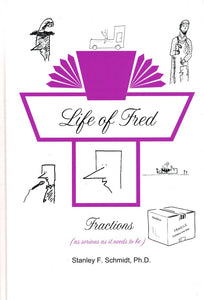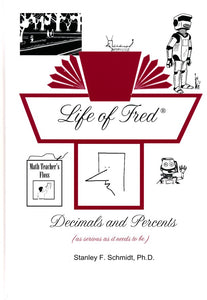# Life of Fred Getting Ready for High School Arithmetic Series: Grades 5-8

Vendor
POLKA DOT PUBLISHING
Regular price
\$38.00
Sale price
\$38.00
Quantity must be 1 or more

Life of Fred Getting Ready for High School Arithmetic Series is designed for students who have completed the elementary & intermediate series books, and are at least in the 5th Grade. The Arithmetic Series cover the introduction to pre-aglgebra.

Once you know:
1. the addition tables (What's 5 + 8?)
2. the subtraction tables (What's 8 - 5?)
3. the multiplication tables (What's 7 times 8?)
4. long division (What's 6231 divided by 93?)

...you are ready to start Life of Fred Fractions, the first book in the Arithmetic series. This book goes far beyond basic fractions so you probably do need it even if you have already learned a bit about basic fractions.

After completing Life of Fred Fractions, go on to the Decimals and Percents Book. Again, this book teaches much more than decimals and percents!

Complete these two books, you are ready for pre-algebra!

There are two books in Arithmetic Series, available individually or in a set:

1. Life of Fred: Fractions (Sample Pages)

This book lays the first half of the foundation for pre-algebra including . . .

• Less Than
• Billion
• Cardinal and Ordinal Numbers
• Savings and Expenses
• Definition of a Fraction
• Sectors
• Comparing Fractions
• Reducing Fractions
• Common Denominators
• Roman Numerals
• Least Common Multiples
• Improper Fractions
• Lines of Symmetry
• Division by Zero
• Circumference
• Multiplying Mixed Numbers
• Commutative Law
• Canceling
• Definition of a Function
• Area
• Unit Analysis
• Division of Fractions
• Geometric Figures
• and much more . . .

2. Life of Fred: Decimals and Percents (Sample Pages)

This book covers second half of the introduction to pre-algebra including . . .

• Number Systems
• Multiplying by Ten
• Functions and Inverse Functions
• Pi
• Multiplying Decimals
• Whole Numbers
• Squaring a Number
• Subtracting Mixed Units
• Sets and Subsets
• Union and Intersection of Sets
• Rules of Divisibility
• Division of Decimals
• Repeating Decimals
• Bar Graphs
• Prime and Composite Numbers
• Consecutive Numbers
• The Goldbach Conjecture
• Area of a Circle
• Dollars vs. Cents
• Pie Charts
• Conversion between Percents/ Fractions/Decimals
• 40% of 15
• 30% off
• Distance Equals Rate Times Time
• 15% more
• Area of a Triangle
• Square Roots
• Area of a Parallelogram
• 13 Is What Percent of 52
• Ratio
• Ordered Pairs
• Graphing
• Negative Numbers
• Elapsed Time
• Probability
• And more . . .